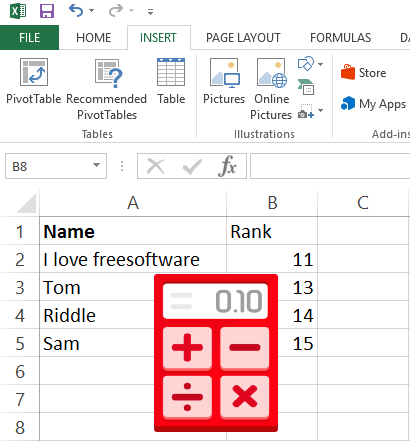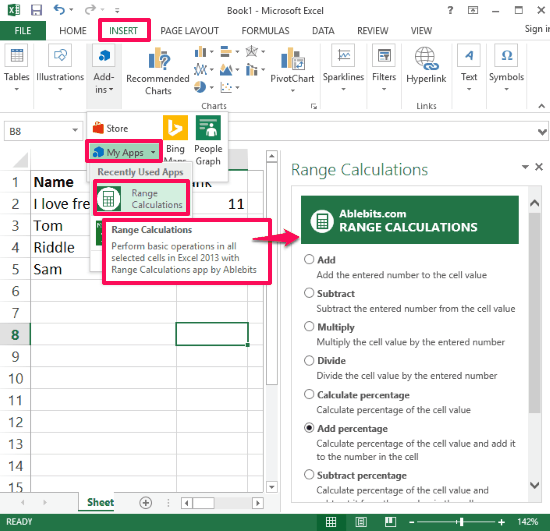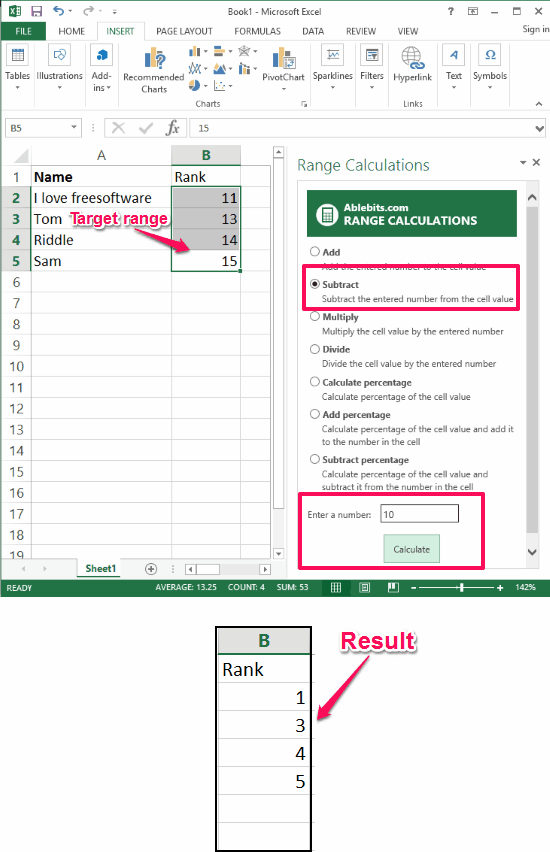Editor Ratings:
User Ratings:
[Total: 0 Average: 0]

This tutorial explains how to do arithmetic operations on a range in Excel. There is an addin for Excel called Range Calculations that can do this. Using the Range Calculations addin, you can easily add, subtract, multiply, a desired range in an Excel sheet with the specified number. Also, it doesn’t immediately change the data in sheet, it first asks you to confirm the operation that is going to be applied to a range. However, the changes made by this addin are not reversible.

If you often make frequent changes in an Excel sheet, but hate time consuming process to change data in cells one by one, then this tutorial will help you. In the tutorial below I have listed an Excel addin that will do that for you. And after going through this tutorial, you will be able to easily do arithmetic operations on a range in Excel.Now, you have a basic idea of what this addin actually does. So to simply cut to the chase, let’s find out what it takes to do arithmetic operations on a range in Excel.

## How To Do Arithmetic Operations On A Range In Excel?

Range Calculations is quite a nice addin to do arithmetic operations on a range in Excel. After using this free addin, you will be easily able to do add, subtract, multiply, divide, like arithmetic operations on a selected range in an Excel sheet. It’s pretty easy to use this addin; you only need Excel 2013 or later installed on your PC.

Follow these simple steps to get started with the Range Calculations addin for Excel.

Step 2: After adding it in Excel you can start using it. Open any Excel sheet and invoke it. To do this Navigate to Insert > My Apps > Range Calculation tab. You will see its interface from the right side of the Excel.Step 3: Now, select a range from the Excel sheet and choose what operation you want to perform in the selected range from its interface. After that, type a number in the text box which is given on its interface. In my case I have subtracted 10 from all the numbers of column B. You can see the following screenshot.So, in this way you can easily do an arithmetic operation on a range in Excel. Range Calculations addin makes it pretty easy to do that. However, the changes that it made are not reversible. So, it is advisable to have a backup copy of the data if it is essential to you.

Also see:

## My Final Verdict

In the tutorial above, I have demonstrated how to do arithmetic operations on a range in Excel. Using the range Calculations addin, you can easily add, subtract, multiply, divide, all the cells of a selected range with the number that you specify. After specifying what operation has to be applied on a certain range, it immediately yields the result. So, if you often make changes in an Excel sheet, then this addin will help you.